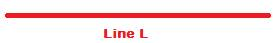## Measurement

Measurement is enunciation of concepts in Numbers.

Measurement is quantification: expressing ideas in numbers.

To measure is to numerate; measurement renders abstruse ideas concrete.

Concepts such as Length, Distance and Height are measured in specific units such as centimetres, metres, kilometres, miles, inches, feet and others.

Measurement of abstract concepts in universally accepted units affords

• enunciation,
• communication and
• comparison

Various systems of measurement have evolved to express in Numbers having Units of Measurement quantities such as Length, Distance, Height, Width, Breadth, Weight, Volume, Temperature, Heat, Cold, Angle, Area, Perimeter, Time, Money and other abstract concepts.

Among the several above, three physical quantities are very commonly encountered by all of us in our daily life. They are

• Length,
• Weight and
• Volume.

Now, read the questions below which we all have asked some time or other:

• How long is the stick?
• How tall are you?

The First Question is a measurement of Length;

The Second, of Distance and

The third, of Height.

Length, Distance and Height are concepts of extension or span or more practically distance between two points.

Length measures distance between two specific points of objects preferably in horizontal position.What is the length of Line L above? May be 5 cms!

Length of a table is a measurement of distance between the two end points of the table.

Length of a stick measures distance between the two tips of the stick.

Likewise, Height measures distance between two specific points of objects in vertical position.

Height of a tower, building, person, and athlete is a measure of distance from bottom to top.

Height of a building is a measure of the distance from the base to its terrace.

In the following solid, length and breadth measure distances of the end points of the respective lines inclined in a horizontal position; height measures the line inclined vertically.

As a concept of measurement, Distance is applied to points very far away from each other, as

• Distance of home from school or office,
• Distance between two places or cities.
• Distance travelled by a bus, car, train or aeroplane.

Units of measurement of length

The Metre is used as the standard unit to measure length.

Around Metre, other units are derived for measuring Length varying from extremely short to very long. The table below lists them:

 1 millimetre 1/1000 metre 1 centimetre 1/100 metre 1 decimetre 1/10 metre 1 metre 1 metre 1 decametre 10 metres 1 hectometre 100 metres 10 hectometres 1kilometre

1000 metres = 1 kilometre

1 kilometre = 1.6 miles or 8/5 miles

1 foot = 12 inches

1 yard = 3 feet = 36 inches = 90 cms

1 metre = 100 cms

1 metre = 10/9 yard